# Quiz:Sliding motion along an inclined plane

1 Consider a situation where$\mu_s = mu_k = \mu$ is the coefficient of static as well as kinetic friction between the block and the incline. What can we say about the normal force between the block (of mass$m$) and the incline as a function of the angle of incline$\theta$ (this is the angle that the incline makes with the horizontal)?
 The magnitude of normal force equals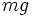$mg$ and is independent of$\theta$ The magnitude of normal force decreases from$mg$ to 0 as$\theta$ increases from 0 to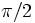$\pi/2$ The magnitude of normal force increases from 0 to$mg$ as$\theta$ increases from 0 to$\pi/2$ The magnitude of normal force increases for$\theta$ increasing from 0 to$\tan^{-1}(\mu)$ and decreases for$\theta$ increasing from$\tan^{-1}(\mu)$ to$\pi/2$ The magnitude of normal force decreases for$\theta$ increasing from 0 to$\tan^{-1}(\mu)$ and increases for$\theta$ increasing from$\tan^{-1}(\mu)$ to$\pi/2$
2 Consider a situation where$\mu_s = mu_k = \mu$ is the coefficient of static as well as kinetic friction between the block and the incline. Once the angle of the incline (denoted$\theta$) exceeds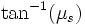$\tan^{-1}(\mu_s)$, the magnitude of downward acceleration$a$ is an increasing function of$\theta$. What is its derivative with respect to$\theta$?$g(\sin \theta + \mu \cos \theta)$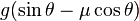$g(\sin \theta - \mu \cos \theta)$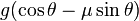$g(\cos \theta - \mu \sin \theta)$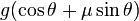$g(\cos \theta + \mu \sin \theta)$